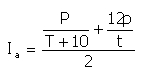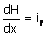﻿ Output > Kennessey's coefficient

FLOWING COEFFICIENT

# FLOWING COEFFICIENT

Not all water that falls into a basin contributes to formation of river,torrent or stream etc.Only the quantity that is not absorbed by the terrain determines the affluence. This quantity depends on factors inherent to the basin’s morphology, its permeability, its vegetal cover etc

There are several methods to determine the flow coefficient , or the ratio between inflow and outflow quantity, referring to a transversal section.

Hydrologic Risk uses Kennessey’s method , applicable particularly to small basins.

Its value is determined on the sum of three interconnected index values: basin’s medium steepness (Ca ), the vegetal cover(Cv ) and its medium permeability (Cp).

Generally, a higher medium steepness contributes to a more intense flowing, reducing the infiltration and evapoperspiration. The presence of a right vegetal cover makes the surface out-flow and the infiltration slower. To this is added the dispersed water by plants perspiration.

A greater permeability contributes to the increasing of the water quantity that infiltrates into the soil, reducing the surface flowing.

To these three factors we have to add the climate of the zone we want to analyse: actually, the flowing coefficient is very influenced by the meteorological events’ distribution over the year, rather than by temperature and rainfall values. Generally, the maximum rainfall associated with high temperatures bring a greater evapoperspiration with consequent decrease of the surface runoff; therefore maximum precipitations contribute to a greater flow.

To take into consideration the climate factor we have to define an aridity index Ia:where:

P = monthly medium inflow;

T = annual medium temperature;

p = inflow of the most arid month;

t = temperature of the most arid month.

With Kennessey’s method we can identify three intervals of the values for the Ia coefficient for every factor (Ca, Cv, Cp):

 Aridity index  Ia Ia< 25 25< Ia < 40 Ia> 40 Permeability  (Cp) Coefficients Very low 0.21 0.26 0.30 Low 0.17 0.21 0.25 Medium 0.12 0.16 0.20 Good 0.06 0.08 0.10 High 0.03 0.04 0.05 steepness (Ca) Coefficients > 35% 0.22 0.26 0.30 35% - 10% 0.12 0.16 0.20 10% - 3.5% 0.01 0.03 0.05 < 3.5% 0.00 0.01 0.03 Vegetation (Cv) Coefficients Rock without vegetation 0.26 0.28 0.30 Pasture 0.17 0.21 0.25 Tilled soil 0.07 0.11 0.15 Forest 0.03 0.04 0.05

Depending of every zone’s aridity index is calculated the contribution of every factor (steepness, permeability and vegetation) considering their distribution on the inner zone of the basin.

Or for every slice of the basin area (basin’s area percentage) the coefficient referring to every factor of the zone is multiplicated (in percentage): every factor will result from the coefficients’ sum as explained before.

The annual medium defflux coefficient will be obtained from the sum of the partial defflux coefficients: Ca, Cv, Cp.

Here is an example of calculation for the partial flowing coefficient depending on the permeability Cp:

Basin’s zone  = 25 Km² with the aridity index < 25

Distributed as follows:

5 Km² are on a high permeability terrain (20% from the total surface);

10 Km² are built on a medium permeability terrain (40% from the total surface);

10 Km² are on a low permeability terrain (40% from the total surface);

Defflux coefficient computation Cp:

0.03 (high permeability coefficient) × 0.20 = 0.0060

0.12 (medium permeability coefficient) × 0.40 = 0.0480

0.21 (low permeability coefficient) × 0.40 = 0.0840

Cp = 0.0060 + 0.0480 + 0.0840 = 0.570

The corresponding process must be followed by the determination of other two flow coefficients. Ca and Cv.